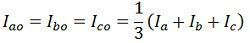# Zero Sequence Current

Definition: The unbalanced current flows in the circuit during the earth fault is known as the zero sequence current or the DC component of the fault current.The zero phase sequence means the magnitude of three phases has zero phase displacement.The three vector lines represent the zero sequence current and it is detected by adding the vector of three phases current. The equation below express the zero-phase sequence current,## Delta-Connected Winding

The delta connected winding is shown in the figure below. The zero sequence current of phases a, b and c are equal in magnitude and in phase with each other. It is circulated in the phase windings of the delta connection as shown in the figure below.The zero sequence currents are produced due to the existence of zero sequence voltage.By KCL at node a, we getSimilarly, by applying KCL at nodes B and C, we haveThe above equation shows that there is no zero sequence current present in the delta connection, because of the absence of the return paths of these current.

Since there is no return path for the zero sequence current in the line, thus the impedance of the circuit becomes infinite.This infinite impedance is shown by the open circuit at point P in the single phase equivalent zero sequence network for a delta connected circuit with zero sequence impedance Z0.But for the zero sequence current, there is the closed path within the delta circuit.This is indicated by connecting the zero sequence impedance Z0 to the zero sequence current.

## Star Connected Winding with Neutral Isolated from Ground

Consider a star connected winding without a neutral return as shown in the figure below.In this case,The above equation shows that the zero sequence current is zero in the three phase three wire system without neutral returns.

## Star Connected Without Neutral Return

The figure below shows the star connected winding with neutral grounded.Here,The above equation shows that for a three-phase grounded system the zero sequence current will flow from phase winding as well as in the lines.